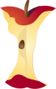Home > Grade Levels > Grade 8 >

## Two Linear Equations in Two Variables#### Aligned To Common Core Standard:

Grade 8 Number System- 8.EE.C.8

### Printable Worksheets And Lessons

• Lines Intersection Step-by-Step Lesson- Find the equation of the lines and then set them equal to each other.

• Guided Lesson - I throw some related word problems in this one. I see these types of questions on the tests all the time.

• Guided Lesson Explanation - These types of problems require a good bit of understanding. You might want to review this one with the kids.

• Independent Practice - Find out where all the lines intersect. They take this skill and use it later to find out timing on collisions in Science class around this time.

• Matching Worksheet - These are a really good mix of problems to start to work out with children.

###Answer Keys

View Answer Keys- All the answer keys in one file.

###(Click Here to Upgrade)

#### Homework Sheets

Start with line intersections and then some tough critical thinking problems.

#### Practice Worksheets

This is one of the more difficult topics for students at this level.

#### Math Skill Quizzes

I threw a review problem on each quiz.

 Quiz 1 Quiz 2 Quiz 3